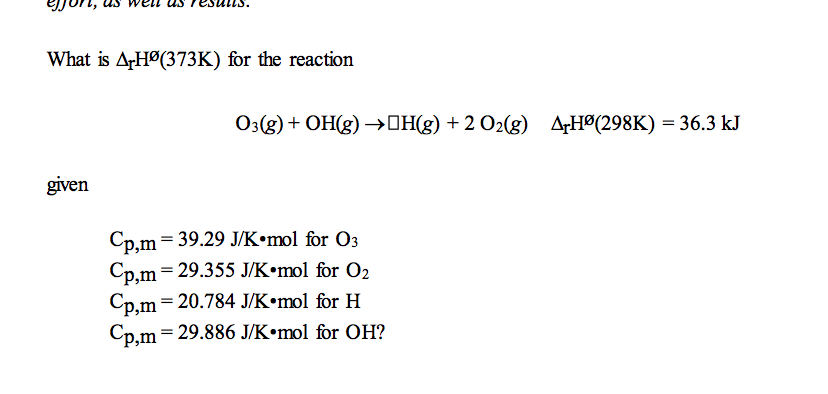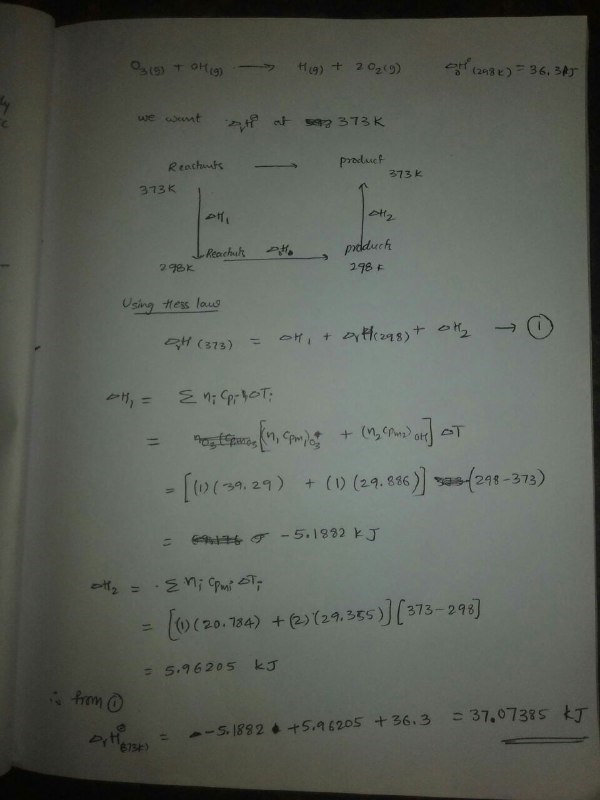Homework Help Question & Answers

What is ArH (373K) for the reaction Odg) + OHig)-> OH(g) + 2 Odg) AHP(298K) = 36.3 kJ given Cp,m-...What is ArH (373K) for the reaction Odg) + OHig)-> OH(g) + 2 Odg) AHP(298K) = 36.3 kJ given Cp,m-39.29 УК.mol for O3 Cp,m= 29.355 J/K·mol for O2 Cp,m-20.7841/K.mol for H Cp.m-29.886 J/K.mol for OH?Add Answer of: What is ArH (373K) for the reaction Odg) + OHig)-> OH(g) + 2 Odg) AHP(298K) = 36.3 kJ given Cp,m-...
More Homework Help Questions Additional questions in this topic.

• Consider the reaction 2H2(g)+ O2(g)>2H20(g) Using standard thermodynamic data at 298K, calculate ...

Need Online Homework Help?

Get FREE EXPERT Answers
WITHIN MINUTES
Related Questions